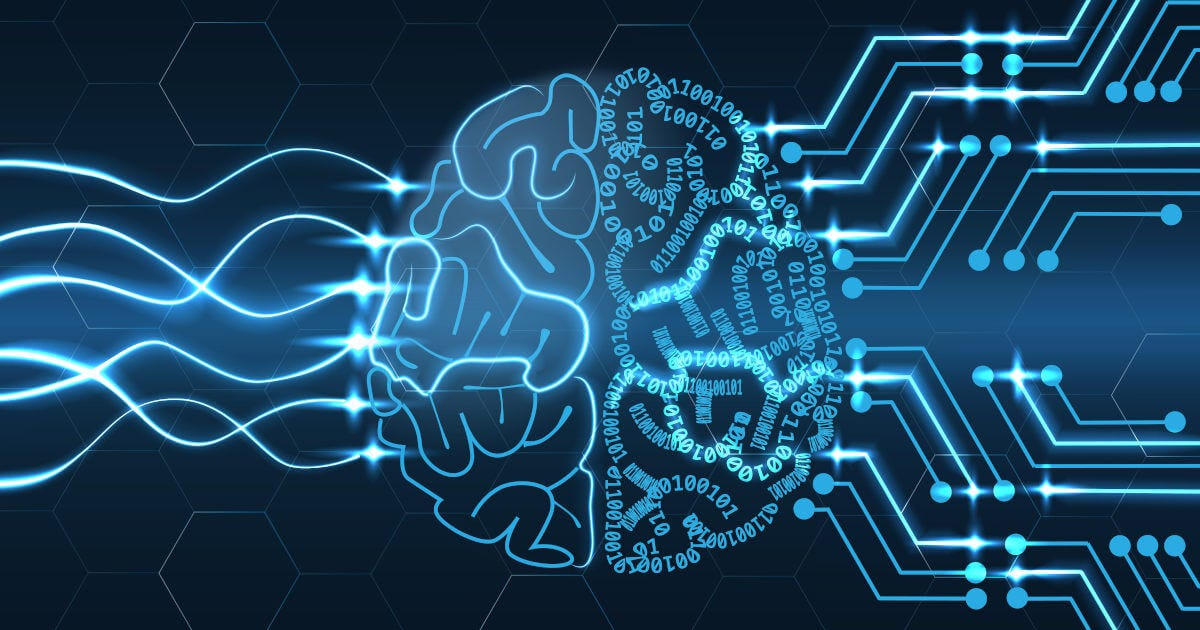# Data Scaling for Machine LearningIt’s possible that you will came across datasets with lots of numerical noise built in, such as lots of variance or differently-scaled data ,the preprocessing solution for that is standardization. Data Scaling for Machine Learning

It’s possible that you will came across datasets with lots of numerical noise built in, such as lots of variance or differently-scaled data ,the preprocessing solution for that is standardization.

Standardization is a preprocessing method used to transform continuous data to make it look normally distributed, in scikit-learn this is often a necessary step, because many models assume that the data you are training on is normally distributed, and if it isn’t, your risk biasing your model, you can standardize your data in different ways, in this article, we’re going to talk about Two popular data scaling methods are normalization and standardization.

It’s also important to note that standardization is a preprocessing method applied to continuous, numerical data, there are a few different scenarios in which you want to standardize your data:

-first, if you are working with any kind of model that uses a linear distance metric or operates on a linear space like K-nearest neighbors, linear regression, or k-means clustering , the model is assuming that the data and features you’re giving it are related in a linear fashion, or can be measured with a linear distance metric.

-second, the case when a feature or features in your dataset have high variance is related to this, this could bias a model that assumes the data is normally distributed, if a feature in your dataset has a variance that’s an order of magnitude or more greater than other features, this could impact the model’s ability to learn from other features in the dataset.

## 15 Machine Learning and Data Science Project Ideas with Datasets

Learning is a new fun in the field of Machine Learning and Data Science. In this article, we’ll be discussing 15 machine learning and data science projects.

## Most popular Data Science and Machine Learning courses — July 2020

Most popular Data Science and Machine Learning courses — August 2020. This list was last updated in August 2020 — and will be updated regularly so as to keep it relevant

## Exploratory Data Analysis is a significant part of Data Science

You will discover Exploratory Data Analysis (EDA), the techniques and tactics that you can use, and why you should be performing EDA on your next problem.

## PyTorch for Deep Learning | Data Science | Machine Learning | Python

PyTorch for Deep Learning | Data Science | Machine Learning | Python. PyTorch is a library in Python which provides tools to build deep learning models. What python does for programming PyTorch does for deep learning. Python is a very flexible language for programming and just like python, the PyTorch library provides flexible tools for deep learning.

## Why You Should Learn R — Learn Data Science with Dataquest

Why should you learn R programming when you're aiming to learn data science? Here are six reasons why R is the right language for you.